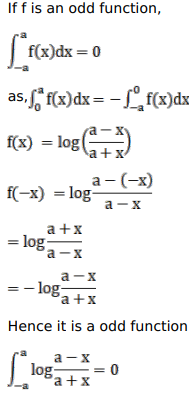# Mark against the correct answer in the following:

Question:

Mark $(\sqrt{)}$ against the correct answer in the following:

$\int_{-a}^{a} \log \left(\frac{a-x}{a+x}\right) d x=?$

A. $2 a$

B. $a$

C. 0

D. 1

Solution: